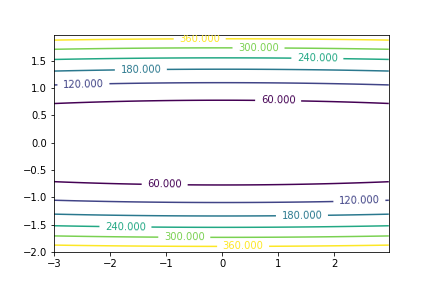$$\DeclareMathOperator*{\argmin}{{arg\,min}} \DeclareMathOperator*{\argmax}{{arg\,max}} \DeclareMathOperator*{\minimize}{{minimize}}$$

# Introduction to optimization algorithms

## Mathematical Optimization

Mathematical Optimization is the branch of mathematics that aims to solve the problem of finding the elements that maximize or minimize a given function. Its importance stems from the fact that many problems in engineering and machine learning can be cast as mathematical optimization problems. For example, in a spam detection filter we might aim to find the system that minimizes the number of misclassified emails. Similarly, when an engineer designs a pipe, we will seek for the design that minimizes cost while respecting some safety constraints. Both are examples that can be modeled as optimization problems.

## Notation

Given an extended real-valued function $f: \RR^p \to \RR$, the general problem of finding the value that minimizes this function is written as follows

\begin{equation}\label{eq:fw_objective} \minimize_{\boldsymbol{x} \in \RR^p} f(\boldsymbol{x}) ~, \end{equation}

In this context, $f$ is the objective function (sometimes referred to as loss function, cost function or energy).

## The rules of the game

Consider the following 2-dimensional optimization problem, with $$\minimize_{x \in \RR^2}\, \underbrace{(1 - x_1)^2 + 100(x_2 - x_1^2)^2}_{= f(x)}~.$$ Since the domain of the objective function is a 2-dimensional space, we can visualize this objective function as an image in 2-D, where the color (darker=lower value) encodes the value of the objective function.

A naive approach is to evaluate the function at a discrete grid and then just select the value that minimize the cost function. However, this approach is very inefficient: the number of function evaluation it requires is exponential in the number of dimensions.

Let $n$ be the grid size in each dimension. Then this approach requires $n^d$ function evaluations. Since we aim to solve problems with large $d$ (in the thousands if not millions), we need more efficient methods.

A more efficient approach instead is to start from an initial guess and iteratively refine the initial guess. The information that to which we have access is the objective function value and its gradient

Not all optimization problems are equal. Knowing your problem enables you to choose the right tool.

### Dimensionality of the problem

The scale of an optimization problem is pretty much set by the dimensionality of the problem, i.e. the number of scalar variables on which the search is performed.

### Convex versus non-convex optimization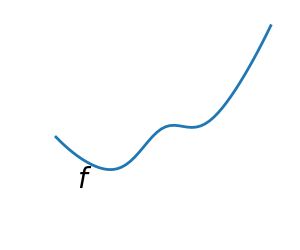A convex function: f is above all its tangents. equivalently, for two point A, B, f(C) lies below the segment [f(A), f(B])], if A < C < B A non-convex function

Optimizing convex functions is typically easier than optimizing non-convex functions. Convex functions have the nice property that the gradient vanishes only at a global optimum

### Smooth and non-smooth problems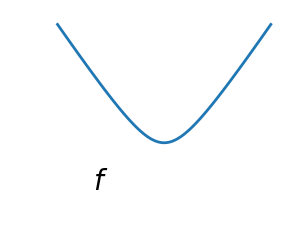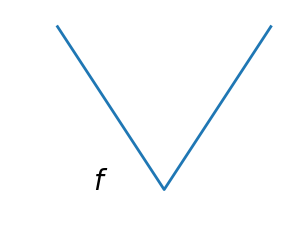A smooth function: The gradient is defined everywhere, and is a continuous function A non-smooth function

Optimizing smooth functions is easier (true in the context of general function, otherwise Linear Programming is an example of methods which deal very efficiently with piece-wise linear functions).

### Noisy versus exact cost functions

 Noisy (blue) and non-noisy (green) functions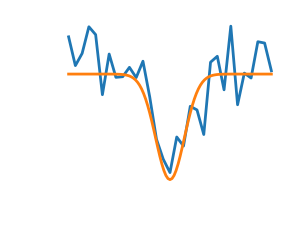In some cases, computing the full objective and gradient is too costly, and we only have access to a noisy estimate of these quantities.

### Constraints

 Optimizations under constraints Here: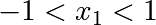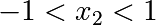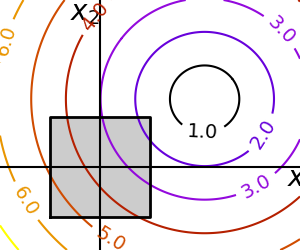### Conditioning

The speed of convergence of most optimization depend on the conditioning of our problem. A well conditioned problem is one in which the curvature is similar among any direction. A badly conditioned problem is one in which the level curves

 Well conditioned problem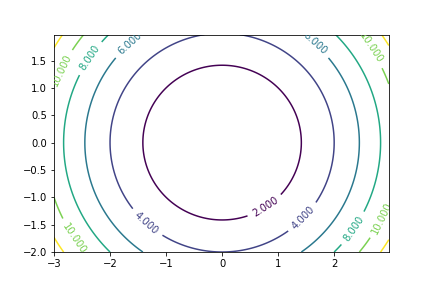Badly conditioned problem